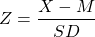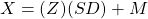# Worksheet 3b.

These guided exercises will give you the opportunity to practice the techniques and apply the concepts you learned in Chapter 3b. You will need some paper, spreadsheet, or a tablet to work on the analyses.

You will need the following formulas to calculate conversions between raw and Z scores.In 1998 information about mean and standard deviation of children’s heights in the US was obtained via the Anthropometric Desk Reference web page. Assume that heights are normally distributed for each age group and sex.

1. Draw a sketch to display the probability that one randomly selected 4-year-old girl has a height less than 102.2 cm. Then use the Z-tables to find this probability. (Mean Height = 102.9cm, SD = 4.3cm)

HINTS:

• Convert raw score into Z score.
• Shade the part of the curve that represents heights less than that Z-score.
• Find the area under the curve that corresponds to that Z-score.
• Remember this distribution is symmetrical. The area under the curve to the right of Z = 2.5 is the same as the area under the curve to the left of Z = -2.5 (for example).

1. Draw a sketch to display the probability that one randomly selected 9-year-old girl has a height between 126 cm and 140 cm. Then use the Z-tables to find this probability. (HINT: You know that the total area under the curve is 100%, so you can subtract off chunks to find remainders.) (Mean Height = 132.2cm, SD = 5.7cm)

1. Draw a sketch to display the 35th percentile of heights for 4-year-old boys. You may use this as a metric to advise families on whether to give their child a break from stimulant medications for ADHD (which can delay growth). Then use the Z-tables to find the 35th percentile and convert it to a raw score. (HINT: The 35th percentile is the score below which 35% of the area under the curve falls). (Mean Height = 101.6cm, SD = 4.0cm)

1. Draw a sketch to display the probability that one randomly selected 4-year-old girl has a height greater than 102.2 cm. Then use the Z-tables to find this probability. (Mean Height = 102.9cm, SD = 4.3cm)

HINTS:

• Convert raw score into Z score.
• Shade the part of the curve that represents heights greater than that Z-score.
• Find the area under the curve that corresponds to that Z-score.
• Remember this distribution is symmetrical. The area under the curve to the right of Z = 2.5 is the same as the area under the curve to the left of Z = -2.5 (for example). Also remember that the total area of the curve adds up to 100%, and half of the curve is 50%.

1. Draw a sketch to display the probability that one randomly selected 4-year-old boy has a height less than 101.6 cm. Then use the Z-tables to find this probability. (Mean Height = 101.6cm, SD = 4.0cm)

1. Draw a sketch to display the probability that one randomly selected 9-year-old girl has a height between 135 cm and 145 cm. Then use the Z-tables to find this probability. (Mean Height = 132.2cm, SD = 5.7cm)

1. Draw a sketch to display the probability that one randomly selected 9-year-old girl has a height between 125.5 cm and 129.5 cm. Then use the Z-tables to find this probability. (Mean Height = 132.2cm, SD = 5.7cm)

1. Draw a sketch to display the 80th percentile of heights for 4-year-old boys. Then use the Z-tables to find the 80th percentile and convert it to a raw score. (Mean Height = 101.6cm, SD = 4.0cm)